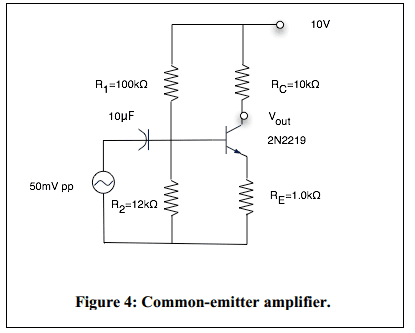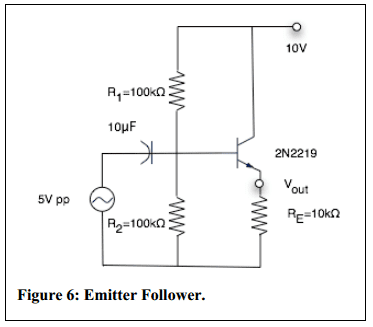# Transistor/Amplifier pre-lab; Inverting vs Non-inverting

## Homework Statement

There are two amplifier circuits in my electronics lab. I know one is inverting and one is not but I can't put my finger on why.

(A) I have to explain why R1 and R2 are about the same for the emitter follower (non-inverting / 'common collector').

I also need to (B) explain why the inverting is an inverting amplifier and (C) why the non-inverting is a non-inverting amplifier.

Common-Emitter (inverting)Common-Collector (non-inverting)## The Attempt at a Solution

(A)
In my notes I have that usually R1≈R2 but my prof. didn't say why. My understanding is that for this type of amplifier you actually don't want a voltage amplification. The use of the amplifier is to go from a weak signal to a strong signal. I would imagine the ratio of these resistors has something to do with that but I can't put my finger on why that is the case. Is it because:

Av=$\frac{ΔV_{out}}{ΔV_{in}}$=$\frac{ΔV_{E}}{ΔV_{B}}$

To make sure that VE and VB are the same we removed the RC resistor and made the other two the same?

(B)/(C)
I read that inverting amplifiers ground their non-inverting source and don't ground their inverting source. The alternative seems to be true for non-inverting amplifiers. This doesn't seem to be clear in the schematic though. Is there some other reason? If not, any suggestions as to how I can view this schematic in that sense?

Thank you!

(A) The emitter follower will only be able to function if the base voltage is between 0 and 10 volts. R1 and R2 are chosen to make that happen, while the input voltage is beteen -5 and +5 volts.

(B/C) those grounded non-inverting/inverting sources happen with opams, and they are not relevant here.

To find out wether an amplifier is inverting:
1 - find out what the base voltage is with no input signal $V_0$
2 - now apply a small input voltage ΔV, so the base voltage is at $V_0 + \Delta V$
3 - now find out what the base current in case 1 and case 2.
4 - same for the collector current in both cases
5. same for the voltage across the collector resistance (common emitter) or emitter resistance (emittor follower)
6. from the results in 5. you can find v-out in both cases. If v_out goes down when ΔV is positive, you have an inverting amplifier

•1 person